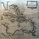Dilihat 1084 kali
Code takes six pairs that are highly correlated to GBPNZD and determines if their ROC's are increasing or decreasing. If a pair has an increasing ROC it is given a 1, if decreasing a -1. The numbers are all added up (this is similar to a count for counting cards in blackjack). If the count goes positive the strategy enters a long position, if negative a short position.

Code is tuned for GBPNZD for 1HR chart. Returns \$97 on an initial balance of \$100 (if I am reading Tradingview Tester correctly)
*** Should work for GBPJPY , its has the same correlated pairs

```//@version=2
strategy("GBPNZD ROC RF count",default_qty_type = strategy.percent_of_equity, default_qty_value = 100,currency="USD",initial_capital=100)

l=input(title="ROC Length",defval=40)
s = input(title="Smoother", type=integer,defval=26, minval=1)

p0 = "FX_IDC:gbpaud"
p1 = "gbpsgd"
p3 = "FX_IDC:eurgbp"
p6 = "gbpjpy"
p7 = "gbpnzd"
p8 = "gbpusd"
s0= security(p0, period, close)
s1= security(p1, period, close)
s3= security(p3, period, close)
s6= security(p6, period, close)
s7= security(p7, period, close)
s8= security(p8, period, close)
r0 = roc(s0, l)
r1 = roc(s1, l)
r3 = roc(s3, l)
r6 = roc(s6, l)
r7 = roc(s7, l)
r8 = roc(s8, l)
c0=iff( r0 > 0,1,0)
cc0=iff( (r0<  0),-1,0)
c1=iff( r1 > 0,1,0)
cc1=iff( (r1<  0),-1,0)
c3=iff( r3 > 0,-1,0)
cc3=iff( (r3 < 0),1,0)
c6=iff( r6 > 0,1,0)
cc6=iff( (r6<  0),-1,0)
c7=iff( r7 > 0,1,0)
cc7=iff( (r7 < 0),-1,0)
c8=iff( r8 > 0,1,0)
cc8=iff( (r8  <0),-1,0)
count = sma(c3+cc3+c0+cc0+c1+c6+cc1+cc6+c7+cc7+c8+cc8,5)
cs=sma(count,s)

plot(cs,color=yellow)
hline(0,color=aqua,linewidth=1,editable=true)

inpTakeProfit = input(defval = 0, title = "Take Profit", minval = 0)
inpStopLoss = input(defval = 0, title = "Stop Loss", minval = 0)
inpTrailStop = input(defval = 0, title = "Trailing Stop Loss", minval = 0)
inpTrailOffset = input(defval = 0, title = "Trailing Stop Loss Offset", minval = 0)
useTakeProfit = inpTakeProfit >= 1 ? inpTakeProfit : na
useStopLoss = inpStopLoss >= 1 ? inpStopLoss : na
useTrailStop = inpTrailStop >= 1 ? inpTrailStop : na
useTrailOffset = inpTrailOffset >= 1 ? inpTrailOffset : na

longCondition = crossover(cs,0)
shortCondition = crossunder(cs,0)
strategy.entry(id = "Long", long=true, when = longCondition)
strategy.close(id = "Long", when = shortCondition)
strategy.entry(id = "Short", long=false, when = shortCondition)
strategy.close(id = "Short", when = longCondition)
strategy.exit("Exit Long", from_entry = "Long", profit = useTakeProfit, loss = useStopLoss, trail_points = useTrailStop, trail_offset = useTrailOffset)
strategy.exit("Exit Short", from_entry = "Short", profit = useTakeProfit, loss = useStopLoss, trail_points = useTrailStop, trail_offset = useTrailOffset)```

## KomenCarlORourke
@CarlORourke, I may. I dropped tradingview strats a long time ago. At the time I could not delete strats I had posted.
Balas